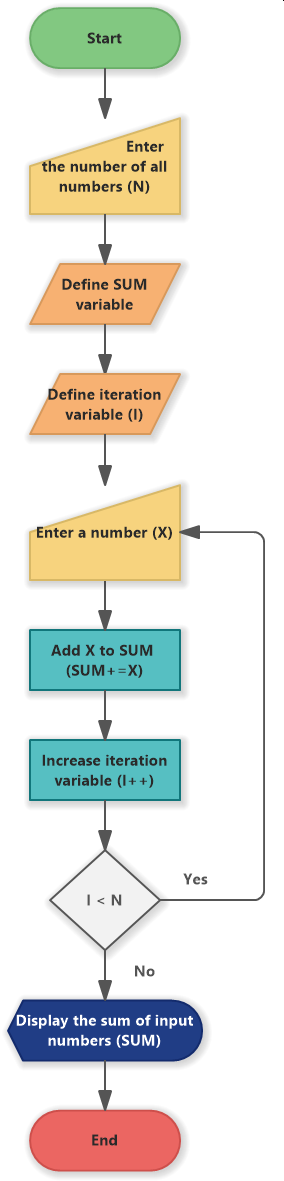# Sum of N Numbers (Flowchart)

An example of a flowchart that shows an algorithm of summing N numbers that were entered by a user. The example shows the usage of various types of flowchart symbols.

## Flowchart for Summing of N Numbers

A flowchart example for a simple algorithm, that receive N numbers from a user input, sum them and display the sum on the output.

The flowchart consists of the following steps:

• Enter the number of all numbers (N)
• Define SUM variable
• Define interation variable (I)
• Enter a number (X)
• Add X to SUM (SIM+=X)
• Increase iteration variable (I++)
• Decision for I < N with two branches - yes and no
• Display the sum of input numbers (SUM)Sum of N numbers (Flowchart)

Download the flowchart to calculate sum of n numbers. You can open and edit it using our flowchart maker.

Sum of N Numbers (Flowchart)